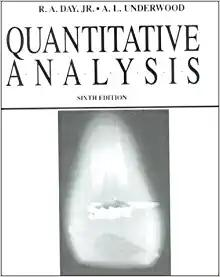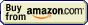# Titrations## Titration » Back titration

Balancer and stoichiometry calculator

operating systems:
XP, Vista, 7, 8, 10

single user license price:
€24.95 - approximately \$25

By clicking Buy Now! you will continue to the FastSpring checkout page where payment will be taken, and your order fulfilled by FastSpring, our trusted reseller, and Merchant of Record.

30-day money back guarantee!

Sometimes it is not possible to use standard titration methods. For example the reaction between determined substance and titrant can be too slow, or there can be a problem with end point determination.

In such situations we can often use a technique called back titration. In back titration we use two reagents - one, that reacts with the original sample (lets call it A), and second (lets call it B), that reacts with the first reagent. How do we proceed? We add precisely measured amount of reagent A to sample and once the reaction ends we titrate excess reagent A left with reagent B. Knowing initial amount of reagent A and amount that was left after the reaction (from titration) we can easily calculate how much reagent A was used for the first reaction.

1.435 g sample of dry CaCO3 and CaCl2 mixture was dissolved in 25.00 mL of 0.9892 M HCl solution. What was CaCl2 percentage in original sample, if 21.48 mL of 0.09312 M NaOH was used to titrate excess HCl?

During titration 21.48×0.09312=2.000 mmole HCl was neutralized. Initially there was 25.00×0.9892=24.73 mmole of HCl used, so during CaCO3 dissolution 24.73-2.000=22.73 mmole of acid reacted. As calcium carbonate reacts with hydrochloric acid 1:2 (2 moles of acid per 1 mole of carbonate), original sample contained 22.73/2=11.37 mmole of CaCO3, or 1.137 g (assuming molar mass of CaCO3 is 100.0 g). So original sample contained 1.137/1.435×100%=79.27% CaCO3 and 100.0-72.27%=20.73% CaCl2.

Direct complexometric Al3+ determination is difficult, as Al3+ reacts with EDTA very slowly. To solution containing some unknown amount of Al3+ cations 50.00 mL of 0.05000 M EDTA solution was added. After 30 minutes excess EDTA was titrated with 0.04875 M Zn2+ solution. What was the amount of Al3+ if 17.58 mL of titrant was used?

EDTA reacts with both Al3+ and Zn2+ in 1:1 ratio. There was 50.00×0.05000=2.500 mmole of EDTA used, and 17.58×0.04875=0.8569 mmole was found to be left. Thus there was 2.500-0.8569=1.643 mmole of Al3+ in the sample.

Page was last modified on July 19 2009, 10:31:43.

titration at www.titrations.info © 2009 ChemBuddy